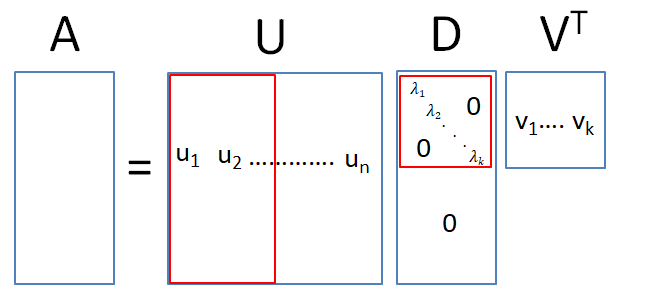Here’s a fun take on the OLS that I picked up from The Elements of Statistical Learning. It applies the Singular Value Decomposition, also known as the method used in principal component analysis, to the regression framework.

### Singular Vector Decomposition (SVD)

First, a little background on the SVD. The SVD could be thought of as a generalisation of the eigendecomposition. An eigenvector v of matrix $$\mathbf{A}$$ is a vector that is mapped to a scaled version of itself: $\mathbf{A}v = \lambda v$ where $$\lambda$$ is known as the eigenvalue. For a full rank matrix (this guarantees orthorgonal eigenvectors), we can stack up the eigenvalues and eigenvectors (normalised) to obtain the following equation: \begin{aligned} \mathbf{A}\mathbf{Q} &= \mathbf{Q}\Lambda \\ \mathbf{A} &= \mathbf{Q}\Lambda\mathbf{Q}^{-1} \end{aligned} where $$\mathbf{Q}$$ is an orthonormal matrix.

For the SVD decomposition, $$\mathbf{A}$$ can be any matrix (not square). The trick is to consider the square matrices $$\mathbf{A}'\mathbf{A}$$ and $$\mathbf{A}\mathbf{A}'$$. The SVD of the $$n \times k$$ matrix $$\mathbf{A}$$ is $$\mathbf{U}\mathbf{D}\mathbf{V}'$$, where $$\mathbf{U}$$ is a square matrix of dimension $$n$$ and $$\mathbf{V}$$ is a square matrix of dimension $$k$$. This implies that $$\mathbf{A}'\mathbf{A} = \mathbf{V}\mathbf{D}^{2}\mathbf{V}$$ and $$\mathbf{V}$$ can be seen to be the eigenvalue matrix of that square matrix. Similarly, the eigenvectors of $$\mathbf{A}\mathbf{A}'$$ forms the columns of $$\mathbf{U}$$ while $$\mathbf{D}$$ is the square root of the eigenvalues of either matrix.

In practice, there is no need to calculate the full set of eigenvectors for both matrices. Assuming that the rank of $$\mathbf{A}$$ is k, i.e. it is a long matrix, there is no need to find all n eigenvectors of $$\mathbf{A}\mathbf{A}'$$ since only the elements of the first k eigenvalues will be multiplied by non-zero elements. Hence, we can restrict $$\mathbf{U}$$ to be a $$n \times k$$ matrix and let $$\mathbf{D}$$ be a $$k \times k$$ matrix.

Here’s a paint illustration of the dimensions of the matrices produced by the SVD decomposition to illustrate the idea more clearly. The $$n \times k$$ matrix produced by multiplying $$\mathbf{U}$$ and $$\mathbf{D}$$ is equivalent for both the blue (keeping all n eigenvectors) and red (keeping only the relevant k eigenvectors) boxes.### Applying the SVD to OLS

To apply the SVD to the OLS formula, we re-write the fitted values, substituting the data input matrix $$X$$ with its equivalent decomposed matrices:

\begin{aligned} \mathbf{X}\hat{\beta} &= \mathbf{X}(\mathbf{X}'\mathbf{X})^{-1}\mathbf{X}'\mathbf{y} \\ &= \mathbf{U}\mathbf{D}\mathbf{V}'(\mathbf{V}\mathbf{D}'\mathbf{U}'\mathbf{U}\mathbf{D}\mathbf{V}')^{-1}\mathbf{V}\mathbf{D}'\mathbf{U}'\mathbf{y} \\ &= \mathbf{U}\mathbf{D}(\mathbf{D}'\mathbf{D})^{-1}\mathbf{D}\mathbf{U}'\mathbf{y} \\ &= \mathbf{U}\mathbf{U}'\mathbf{y} \end{aligned} where the third to fourth line comes from the fact that $$(\mathbf{D}'\mathbf{D})^{-1}$$ is a $$k \times k$$ matrix with the square root of the eigenvalues on the diagonal, and $$\mathbf{D}$$ is a square diagonal matrix. Here we see that the fitted values are computed with respect to the orthonormal basis $$\mathbf{U}$$.1

1. Doing a QR decomposition will also give a similar set of results, though the orthogonal bases will be different.